﻿ 面向动用计划的车辆装备备件预测研究
«上一篇文章快速检索 高级检索

 智能系统学报2021, Vol. 16Issue (6): 1064-1072  DOI: 10.11992/tis.2020120260

### 引用本文LI Fengyue, QI Xiaogang, BAN Liming, et al. Vehicle maintenance spare-part prediction for equipment use plan[J]. CAAI Transactions on Intelligent Systems, 2021, 16(6): 1064-1072. DOI: 10.11992/tis.202012026.### 文章历史

1. 西安电子科技大学 数学与统计学院，陕西 西安 710000;
2. 中国人民解放军32272部队，甘肃 兰州 730000

Vehicle maintenance spare-part prediction for equipment use plan
LI Fengyue 1, QI Xiaogang 1, BAN Liming 2, LI Jianhua 2, SUO Wenkai 2
1. School of Mathematics and Statistics, Xidian University, Xi’an 710000, China;
2. 32272 Group of PLA, Lanzhou 730000, China
Abstract: Aiming at the consumption characteristics of vehicle equipment spare parts under the use plan, two forecast optimization problems of spare-part consumption and inventory control are studied. First, the preventive and repairable maintenance of vehicle equipment is considered for the use plan period, and the consumption of equipment maintenance spare parts under the fixed schedule and random natural failure maintenance is predicted. Subsequently, based on the characteristics of the spare parts inventory inspection method, the joint replenishment inventory control model is established based on a regular inspection strategy. According to the structural characteristics of the model, the limits of decision variables are determined, and the multipopulation location upgrade method is used to improve the fruit fly optimization algorithm (FOA). The simulation results show that the improved FOA has good solving efficiency. The proposed optimization method can provide a decision-making basis for the optimization of vehicle maintenance support resources.
Key words: vehicle equipment    spare parts prediction    using plan    consumption    regular inspection    inventory control    multi-population    fruit fly optimization algorithm

1 车辆装备备件消耗量模型 1.1 车辆装备备件消耗过程分析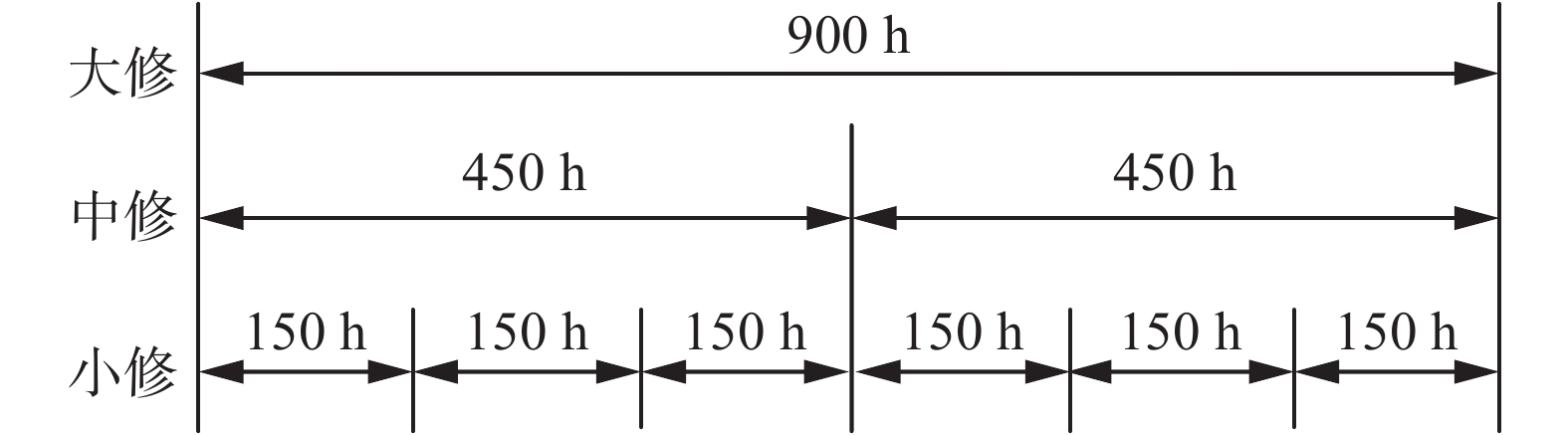Download: 图 1 维修间隔期示意 Fig. 1 Schematic diagram of maintenance intervals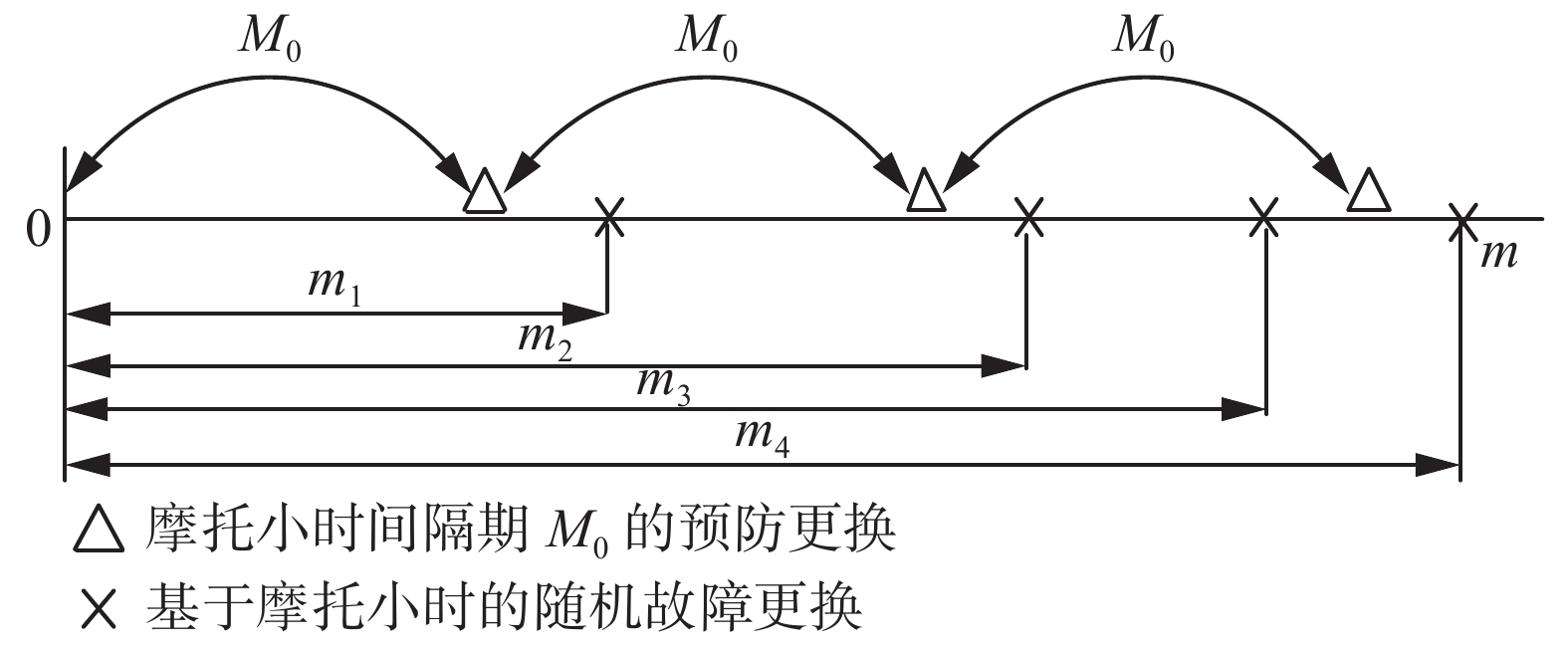Download: 图 2 车辆装备备件更换策略 Fig. 2 Vehicle equipment spare-part replacement strategy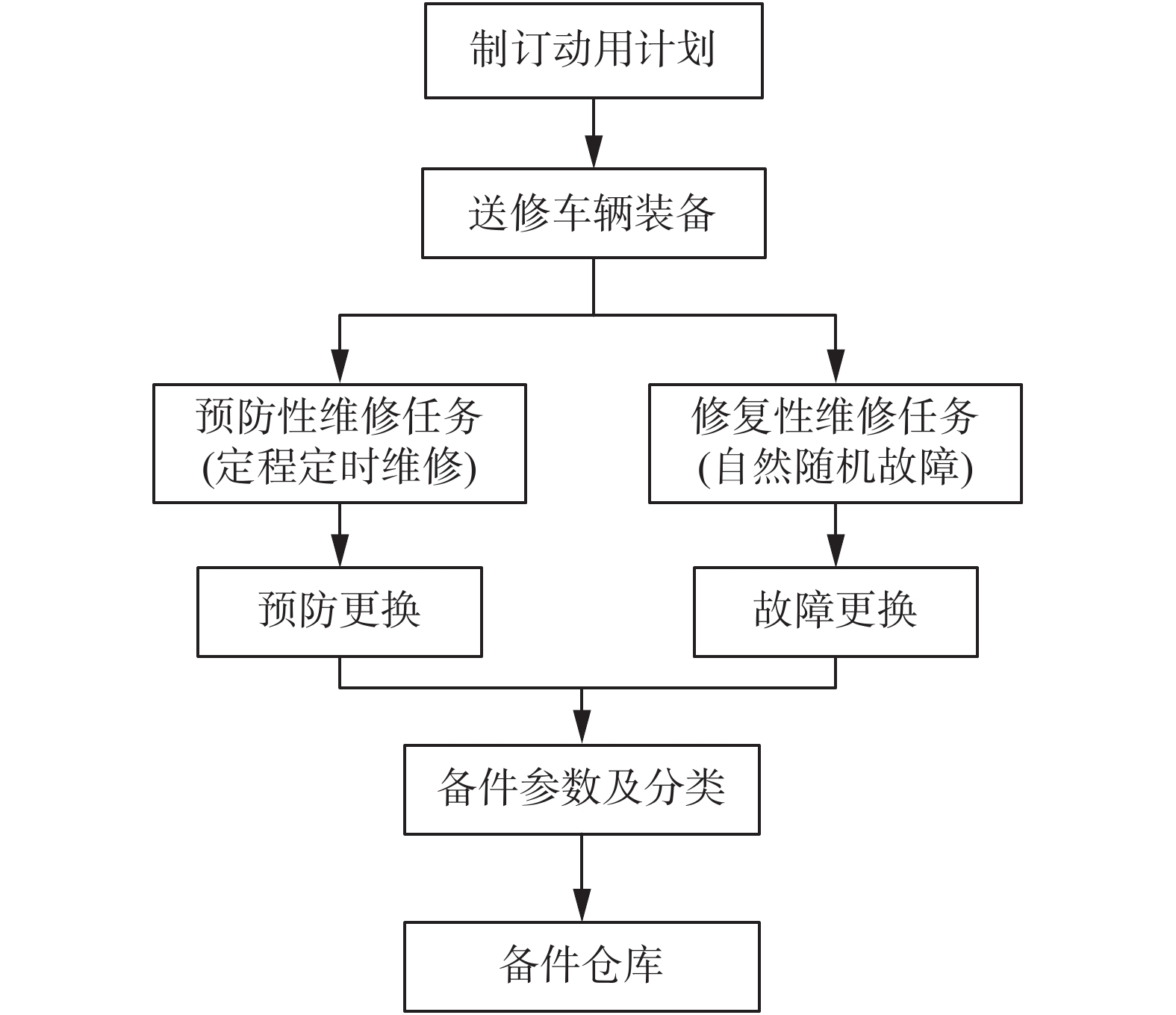Download: 图 3 备件消耗过程 Fig. 3 Spare-part consumption process
1.2 车辆装备备件消耗量模型表 1 基本符号 Tab.1 Basic symbol

1)定时定程维修备件消耗量

 ${Z_j} = \sum\limits_{k = 1}^N {\left\lfloor {\frac{{{M_k} - {M_{jk}} + {{\rm{EM}}_k}}}{{{{\rm{IM}}_j}}}} \right\rfloor }$ (1)

 ${D'_i} = \sum\limits_{\forall f} {\sum\limits_{j = 1}^3 {{Z_j} \cdot } {\gamma _{jf}} \cdot {c_{fi}}}$ (2)

2)随机故障维修备件消耗量

 ${H_f} = \sum\limits_{\forall l} {\sum\limits_{\forall k} {\Delta {M_{kl}}{\lambda _l}{\beta _f}} }$ (3)

 ${D''_i} = \sum\limits_{\forall f} {({H_f} \cdot {c_{fi}})}$ (4)

3)动用计划下备件消耗量模型

 $\begin{array}{l} {D_i} = {D'_i} + {D''_i} = \displaystyle\sum\limits_{\forall f} {\displaystyle\sum\limits_{j = 1}^3 {{Z_j} \cdot } {\gamma _{jf}} \cdot {c_{fi}}} + \displaystyle\sum\limits_{\forall f} {({H_f} \cdot {c_{fi}})} \end{array}$ (5)
2 车辆装备备件库存控制模型

$N$ 种装备备件中，第 $i$ 种备件的订购量 ${Q_i}$

 ${Q_i} = {D_i}{k_i}T, \;\;i = 1,2, \cdots, N$ (6)

 ${C_h} = \frac{1}{2}\sum\limits_{i = 1}^N {{Q_i}{h_i}} = {{\left( {\sum\limits_{i = 1}^N {{k_i}T{D_i}} } \right)} / 2}$ (7)

 ${C_r} = \left( {{R / T}} \right) + \sum\limits_{i = 1}^N {\left( {{{{r_i}} / {{k_i}T}}} \right)}$ (8)

 $\begin{array}{l} C(T,K) = {C_h} + {C_r} = \displaystyle\sum\limits_{i = 1}^N {\dfrac{{{k_i}T{D_i}}}{2}} + \dfrac{R}{T} + \displaystyle\sum\limits_{i = 1}^N {\dfrac{{{r_i}}}{{{k_i}T}}} \end{array}$ (9)

3 算法设计

3.1 界限确定

 ${T^*}(K) = \sqrt {{{2\left( {R + \sum\limits_{i = 1}^N {\frac{{{r_i}}}{{{k_i}}}} } \right)} / {\sum\limits_{i = 1}^N {{k_i}{D_i}{h_i}} }}}$ (10)

 $C(K) = \sqrt {2(R + \sum\limits_{i = 1}^N {\frac{{{r_i}}}{{{k_i}}}} )\sum\limits_{i = 1}^N {{D_i}{k_i}{h_i}} } {\text{ }}$ (11)

${k_i}$ 为大于零的整数，所以显然可以设置下限 ${k_{{\rm{LB}}i}}$ =1。另一方面，通过 $\left( {{{\partial C} / {\partial {k_i}}}} \right) = 0$ 和基本不等式(12)，易得出不等式(13)：

 ${k_i}({k_i} - 1) \leqslant k_i^2 \leqslant {k_i}({k_i} + 1)$ (12)
 ${k_i}({k_i} - 1) \leqslant \frac{{2{r_i}}}{{{D_i}{h_i}{T^2}}} \leqslant {k_i}({k_i} + 1)$ (13)

 ${k_{{\rm{UB}}i}}({k_{{\rm{UB}}i}} - 1) \leqslant \frac{{2{r_i}}}{{{D_i}{h_i}T_{\min }^2}} \leqslant {k_{{\rm{UB}}i}}({k_{{\rm{UB}}i}} + 1)$ (14)

3.2 种群更新方式

1)果蝇“发现者”位置更新方式

 $X_y^{t + 1} = X_y^t + {\text{rand}}({\text{F}}{{\text{R}}_1})$ (15)

2)果蝇“跟随者”位置更新方式

 $X_y^{t + 1} = X_d^{t + 1} + {\text{rand}}\left( {{\text{F}}{{\text{R}}_2}} \right)$ (16)

3)果蝇“反捕食行为”方式

 $X_y^{t + 1} = X\_{\text{axis}} + {\text{rand}}\left( {{\text{F}}{{\text{R}}_3}} \right)$ (17)

1)初始化参数。设置种群规模、最大迭代次数、飞行方向，果蝇各类群体比例。随机初始化果蝇个体位置。

2)计算果蝇的气味浓度值。气味浓度判断值 ${S_y} = {X_y}$ ，将 ${S_y}$ 代入味道浓度判定函数 $F(X)$ 中，本文的判定函数 $F(X)$ 为总成本函数 $C(K)$ 。计算每个个体当前位置的味道浓度值 ${\text{Smel}}{{\text{l}}_y}$ ${\text{Smel}}{{\text{l}}_y} = F({S_y})$

3)对气味浓度值进行排序 ${\text{sort}}({X_i})$ 。记录种群的全局历史最佳位置 $X\_{\text{axis}}$ 和最佳气味浓度值 ${\text{Smellbest}}$ ；根据气味浓度值大小和群体比例参数 $M$ 设置果蝇“发现者”“跟随者”和“反捕食行为”群体。

4)多类种群位置更新。根据式(15)~(17)更新果蝇群体位置。

5)重复步骤2)~4)，直到迭代次数最大，停止迭代并输出结果。

4 仿真与分析 4.1 实例仿真表 2 基础数据参数 Tab.2 Basic data parameters表 3 车辆动用计划 Tab.3 Vehicle usage plan
4.2 仿真分析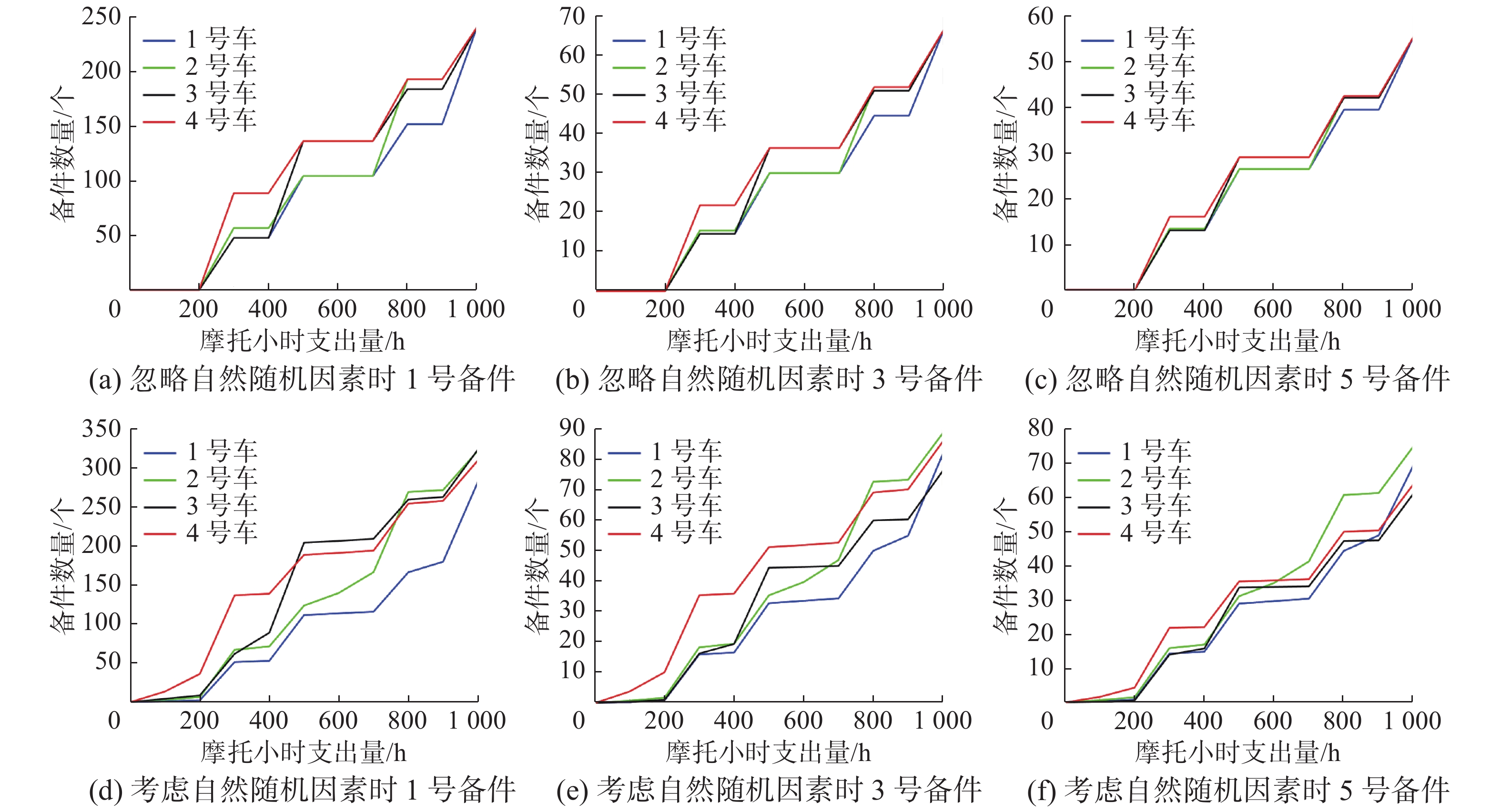Download: 图 4 备件消耗量变化规律 Fig. 4 Spare-part consumption change rule表 4 备件消耗量 Tab.4 Spare-part consumption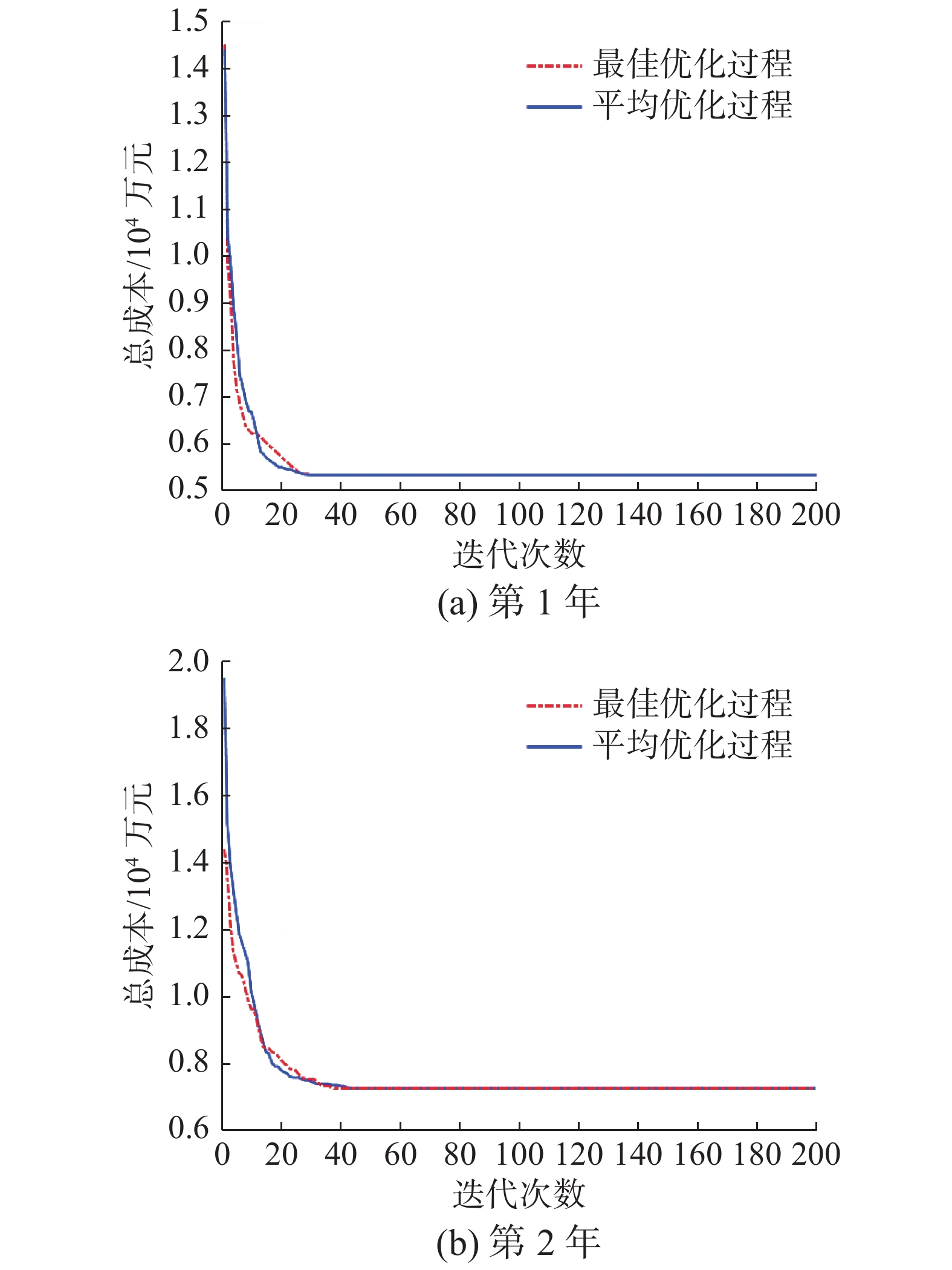Download: 图 5 IFOA收敛过程对比 Fig. 5 Convergence process comparison of IFOA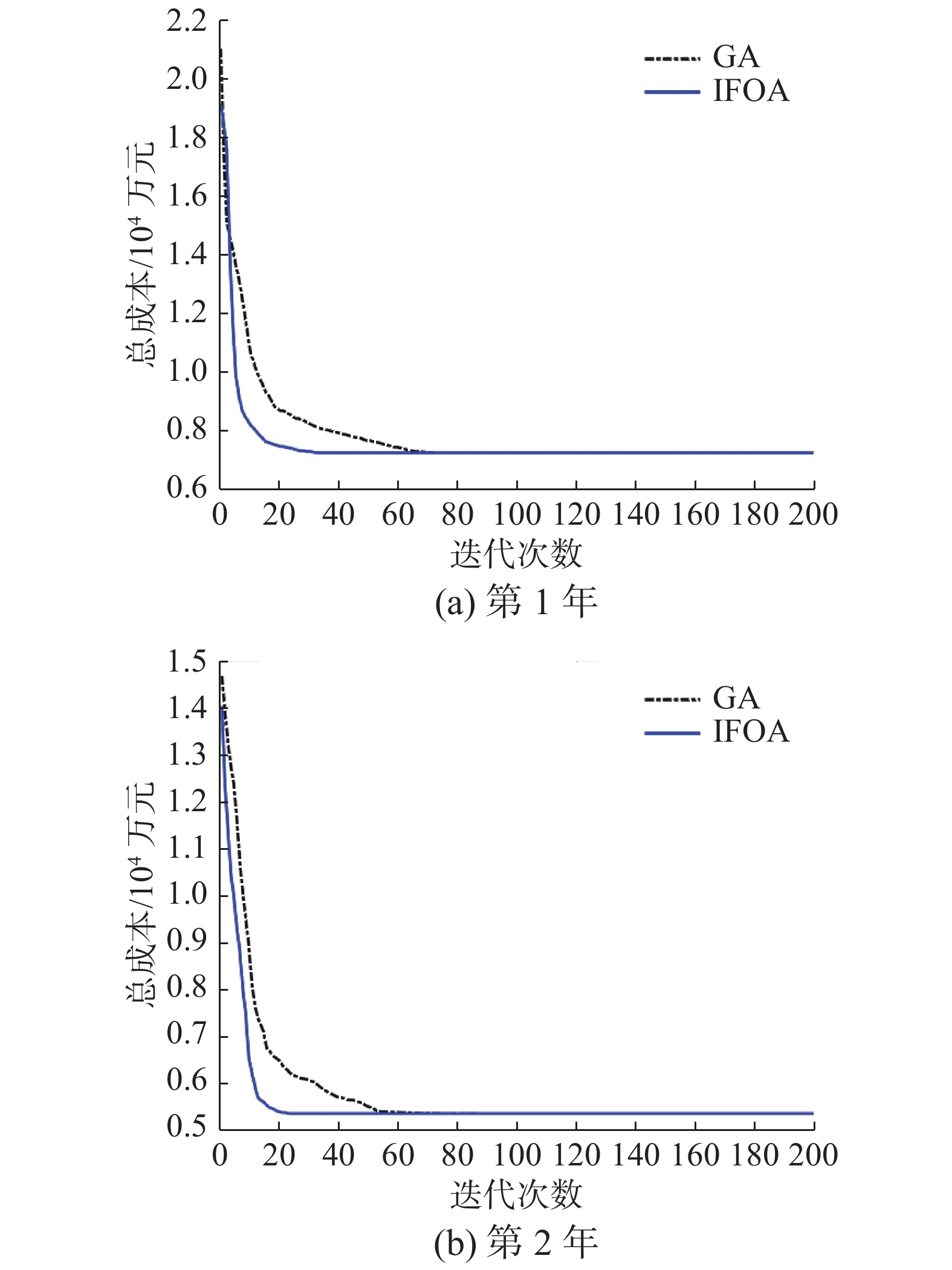Download: 图 6 IFOA与GA收敛过程对比 Fig. 6 Comparison of IFOA and GA convergence processes表 5 第1年库存控制方案 Tab.5 Inventory control plan for the first year表 6 第二年库存控制方案 Tab.6 Inventory control plan for the second year
5 结束语

  杨超, 侯兴明, 廖兴禾, 等. 装备维修保障资源配置文献分析与内容综述[J]. 兵器装备工程学报, 2019, 40(7): 170-175. YANG Chao, HOU Xingming, LIAO Xinghe, et al. Literature analysis and content review of equipment maintenance support resource allocation[J]. Journal of ordnance equipment engineering, 2019, 40(7): 170-175. DOI:10.11809/bqzbgcxb2019.07.034 (0)  高颖杰, 曹继平, 雷宁, 等. 装备维修保障资源配置优化研究综[J]. 火炮发射与控制学报, 2020, 41(3): 102-106. GAO Yingjie, CAO Jiping, LEI Ning, et al. Review of study on equipment maintenance support resource allocation optimization[J]. Journal of gun launch & control, 2020, 41(3): 102-106. (0)  ZHANG Dapeng, LI Fengju, WANG Fengzhong, et al. Research on vehicle equipment warehouse security level optimization based on greedy algorithm[C]//Proceedings of the 2019 2nd International Conference on Information Management and Management Sciences. Chengdu, China, 2019: 118−122. (0)  WANG Jingjing, YIN Hui. Failure rate prediction model of substation equipment based on Weibull distribution and time series analysis[J]. IEEE access, 2019, 7: 85298-85309. DOI:10.1109/ACCESS.2019.2926159 (0)  KUTYŁOWSKA M. Neural network approach for failure rate prediction[J]. Engineering failure analysis, 2015, 47: 41-48. DOI:10.1016/j.engfailanal.2014.10.007 (0)  白润才, 柴森霖, 刘光伟, 等. 基于Mallat算法与ARMA模型的露天矿卡车故障率预测[J]. 中国安全科学学报, 2018, 28(10): 31-37. BAI Runcai, CHAI Senlin, LIU Guangwei, et al. Method for predicting truck's failure rate in open-pit mine based on Mallat algorithm and ARMA model[J]. China safety science journal, 2018, 28(10): 31-37. (0)  GAO Tianshan, GAO Bo. Failure rate prediction of substation equipment combined with grey linear regression combination model[C]//IEEE International Conference on High Voltage Engineering and Application. Chengdu, China, 2016: 1−5. (0)  张浩, 高朝晖. 基于对故障规律统计分析的汽车备件需求预测[J]. 数理统计与管理, 2012, 31(4): 627-632. ZHANG Hao, GAO Zhaohui. Demand forecasting for auto spare parts based on statistical analysis of the failure rules[J]. Journal of applied statistics and management, 2012, 31(4): 627-632. (0)  张建荣, 于永利, 张柳, 等. 维修器材联合订货的库存决策模型[J]. 系统工程与电子技术, 2013, 35(1): 109-112. ZHANG Jianrong, YU Yongli, ZHANG Liu, et al. Inventory decision model of joint ordering policy with maintenance materials[J]. Systems engineering and electronics, 2013, 35(1): 109-112. DOI:10.3969/j.issn.1001-506X.2013.01.18 (0)  CARVAJAL J, CASTAÑO F, SARACHE W, et al. Heuristic approaches for a two-echelon constrained joint replenishment and delivery problem[J]. International journal of production economics, 2020, 220: 107420-107433. DOI:10.1016/j.ijpe.2019.06.016 (0)  杨宇航, 赵建民, 李志忠, 等. 备件管理系统仿真研究[J]. 系统仿真学报, 2004, 16(5): 981-986, 991. YANG Yuhang, ZHAO Jianmin, LI Zhizhong, et al. Research on spares management system with simulation[J]. Journal of system simulation, 2004, 16(5): 981-986, 991. DOI:10.3969/j.issn.1004-731X.2004.05.033 (0)  NAGASAWA K, IROHARA T, MATOBA Y, et al. Applying genetic algorithm for can-order policies in the joint replenishment problem[J]. Industrial engineering and management systems, 2015, 14(1): 1-10. DOI:10.7232/iems.2015.14.1.001 (0)  张建荣, 于永利, 张柳, 等. 基于定周期策略的多品种维修器材联合订购模型[J]. 火力与指挥控制, 2012, 37(9): 112-116. ZHANG Jianrong, YU Yongli, ZHANG Liu, et al. Joint order model of multi-items maintenance spare parts based on periodic policy[J]. Fire control & command control, 2012, 37(9): 112-116. DOI:10.3969/j.issn.1002-0640.2012.09.030 (0)  WANG Yadong, SHI Quan. Improved dynamic PSO-based algorithm for critical spare parts supply optimization under (T, S) inventory policy[J]. IEEE access, 2019, 7: 153694-153709. DOI:10.1109/ACCESS.2019.2948859 (0)  LI Jiying, JI Zhihong. Joint replenishment problem of military aircraft consumptive items under resource constraint[C]//2017 8th International Conference on Mechanical and Aerospace Engineering (ICMAE). Prague, Czech Republic, 2017: 786−790. (0)  ZENG Yurong, PENG Lu, ZHANG Jinlong, et al. An effective hybrid differential evolution algorithm incorporating simulated annealing for joint replenishment and delivery problem with trade credit[J]. International journal of computational intelligence systems, 2016, 9(6): 1001-1015. DOI:10.1080/18756891.2016.1256567 (0)  周云彦, 王建平, 赵聪, 等. 一种装甲装备摩托小时梯次储备的生成与控制方法[J]. 装甲兵工程学院学报, 2012, 26(5): 24-28. ZHOU Yunyan, WANG Jianping, ZHAO Cong, et al. Formation and control method of motor hour echelon storage for a kind of armored equipment[J]. Journal of academy of armored force engineering, 2012, 26(5): 24-28. DOI:10.3969/j.issn.1672-1497.2012.05.005 (0)  赵劲松, 令狐昌应, 贺宇. 战时车辆装备维修任务量测算模型[J]. 军事交通学院学报, 2016, 18(6): 27-31. ZHAO Jinsong, LINGHU Changying, HE Yu. Repair workload measuring model of wartime vehicle equipment[J]. Journal of military transportation university, 2016, 18(6): 27-31. (0)  薛永亮, 陈振林. 基于改进的神经网络对航空发动机故障率预测研究[J]. 计算机测量与控制, 2019, 27(5): 44-47. XUE Yongliang, CHEN Zhenlin. Study on prediction of aeroengine failure rate based on improved neural network[J]. Computer measurement & control, 2019, 27(5): 44-47. (0)  ALIZADEH A, ALAYI N M, FEREIDUNIAN A, et al. A Recurrent neural network approach to model failure rate considering random and deteriorating failures[C]//2021 26th International Computer Conference, Computer Society of Iran. Tehran, Iran, 2021: 1−7. (0)  张铸, 饶盛华, 张仕杰. 一种新型的混沌步长果蝇优化算法[J]. 计算机工程与科学, 2020, 42(4): 755-760. ZHANG Zhu, RAO Shenghua, ZHANG Shijie. A novel fruit fly optimization algorithm with chaotic step[J]. Computer engineering & science, 2020, 42(4): 755-760. DOI:10.3969/j.issn.1007-130X.2020.04.024 (0)  DEL SER J, OSABA E, MOLINA D, et al. HERRERA F. Bio-inspired computation: where we stand and what’s next[J]. Swarm and evolutionary computation, 2019, 48: 220-250. DOI:10.1016/j.swevo.2019.04.008 (0)  SONG Yingjie, WU Daqing, DENG Wu, et al. MPPCEDE: multi-population parallel co-evolutionary differential evolution for parameter optimization[J]. Energy conversion and management, 2021, 228: 113661. DOI:10.1016/j.enconman.2020.113661 (0)  王守娜, 刘弘, 高开周. 一种应用于函数优化问题的多种群人工蜂群算法[J]. 郑州大学学报(工学版), 2018, 39(6): 30-35. WANG Shouna, LIU Hong, GAO Kaizhou. A multiswarm artificial bee colony algorithm for function optimization[J]. Journal of Zhengzhou University ( engineering science edition), 2018, 39(6): 30-35. (0)  SONG Yingjie, WU Daqing, MOHAMED A W, et al. Enhanced success history adaptive DE for parameter optimization of photovoltaic models[J]. Complexity, 2021, 2021: 6660115. (0)  CAI Xing, ZHAO Huimin, SHANG Shifan, et al. An improved quantum-inspired cooperative co-evolution algorithm with muli-strategy and its application[J]. Expert systems with applications, 2021, 171: 114629-114642. DOI:10.1016/j.eswa.2021.114629 (0)  XUE Jiankai, SHEN Bo. A novel swarm intelligence optimization approach: sparrow search algorithm[J]. Systems science & control engineering, 2020, 8(1): 22-34. (0)  石建平, 李培生, 刘国平, 等. 求解约束优化问题的改进果蝇优化算法及其工程应用[J]. 控制与决策, 2021, 36(2): 314-324. SHI Jianping, LI Peisheng, LIU Guoping, et al. Improved fruit fly optimization algorithm for solving constrained optimization problems and engineering applications[J]. Control and decision, 2021, 36(2): 314-324. (0)